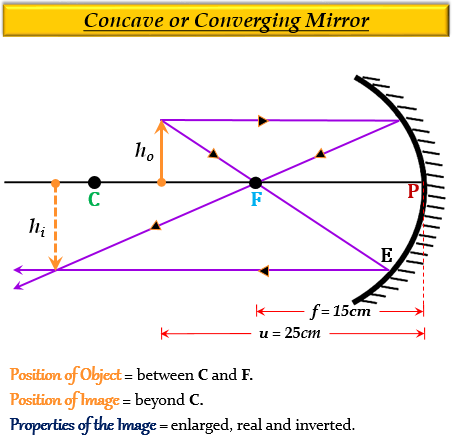# An object 4.0 cm in size, is placed 25.0 cm in front of a concave mirror of focal length 15.0 cm.(i) At what distance from the mirror should a screen be placed in order to obtain a sharp image?(ii) Find the size of the image.(iii) Draw a ray diagram to show the formation of image in this case.

Given:

Height of the object, $h_{1}$ = 4 cm

Distance of the object from the mirror $u$ = $-$25 cm

Focal length of the mirror, $f$ = $-$15 cm

To find: (i) Distance of the image $(v)$ from the mirror.

(ii) Height of the image $(h_2)$.

(i) Solution:

From the mirror formula, we know that-

$\frac{1}{f}=\frac{1}{v}+\frac{1}{u}$

Substituting the given values in the mirror formula we get-

$\frac{1}{(-15)}=\frac{1}{v}+\frac{1}{(-25)}$

$-\frac{1}{15}=\frac{1}{v}-\frac{1}{25}$

$\frac{1}{25}-\frac{1}{15}=\frac{1}{v}$

$\frac{1}{v}=\frac{3-5}{75}$

$\frac{1}{v}=\frac{-2}{75}$

$v=-\frac{75}{2}$

$v=-37.5cm$

Thus, the distance of the image, $v$ is 37.5 cm from the mirror, and the negative sign implies that the image forms in front of the mirror (on the left).

(ii) Solution:

From the magnification formula, we know that-

$m=\frac{{h}_{2}}{{h}_{1}}=-\frac{v}{u}$

Substituting the given values in the magnification formula we get-

$\frac{{h}_{2}}{4}=-\frac{(-37.5)}{(-25)}$

$\frac{{h}_{2}}{4}=-\frac{37.5}{25}$

$\frac{{h}_{2}}{4}=-1.5$

${h}_{2}=4\times {(-1.5)}$

${h}_{2}=-6cm$

Thus, the height of the image $h_2$ is 6 cm, and the negative sign implies that the image forms below the principal axis (downwards).

(iii) The ray diagram showing the formation of the image in this case is-